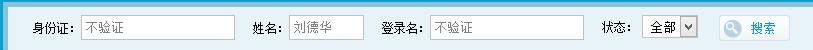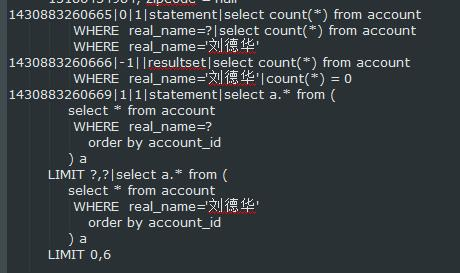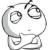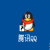# mybatis+spring搭建的web项目，在输入中文参数进行where查询时无法得到结果

jolly878 发布于 2015/05/06 11:48

<select id="accountRows" parameterType="com.future.entity.page.AccountPage" resultType="Integer">
select count(*) from account
<where>
<if test="idcard_no!=null &amp;&amp; idcard_no.length()!=0">
and idcard_no=#{idcard_no,jdbcType=VARCHAR}
</if>
<if test="real_name!=null &amp;&amp; real_name.length()!=0">
and real_name=#{real_name,jdbcType=VARCHAR}
</if>
</if>
<if test="status!=null &amp;&amp; status.length()!=0">
and status=#{status,jdbcType=VARCHAR}
</if>
</where>
</select>这是sql拦截的截图

00<if test="login_name!=null &amp;&amp; login_name.length()!=0">
and login_name like '%$login_name$%'
</if>

0<if test="login_name!=null &amp;&amp; login_name.length()!=0">
</if>